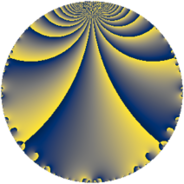# Properties

 Label 37.2.bLevel $37$ Weight $2$ Character orbit 37.b Rep. character $\chi_{37}(36,\cdot)$ Character field $\Q$ Dimension $2$ Newform subspaces $1$ Sturm bound $6$ Trace bound $0$

# Related objects

## Defining parameters

 Level: $$N$$ $$=$$ $$37$$ Weight: $$k$$ $$=$$ $$2$$ Character orbit: $$[\chi]$$ $$=$$ 37.b (of order $$2$$ and degree $$1$$) Character conductor: $$\operatorname{cond}(\chi)$$ $$=$$ $$37$$ Character field: $$\Q$$ Newform subspaces: $$1$$ Sturm bound: $$6$$ Trace bound: $$0$$

## Dimensions

The following table gives the dimensions of various subspaces of $$M_{2}(37, [\chi])$$.

Total New Old
Modular forms 4 4 0
Cusp forms 2 2 0
Eisenstein series 2 2 0

## Trace form

 $$2 q - 2 q^{3} - 4 q^{4} + 6 q^{7} - 4 q^{9} + O(q^{10})$$ $$2 q - 2 q^{3} - 4 q^{4} + 6 q^{7} - 4 q^{9} + 8 q^{10} - 6 q^{11} + 4 q^{12} - 8 q^{16} - 6 q^{21} + 2 q^{25} + 24 q^{26} + 10 q^{27} - 12 q^{28} - 8 q^{30} + 6 q^{33} - 8 q^{34} + 8 q^{36} - 2 q^{37} - 24 q^{38} - 6 q^{41} + 12 q^{44} - 16 q^{46} + 6 q^{47} + 8 q^{48} + 4 q^{49} + 18 q^{53} + 16 q^{58} - 12 q^{63} + 16 q^{64} - 24 q^{65} - 24 q^{67} + 24 q^{70} - 6 q^{71} + 18 q^{73} - 24 q^{74} - 2 q^{75} - 18 q^{77} - 24 q^{78} + 2 q^{81} + 18 q^{83} + 12 q^{84} + 8 q^{85} + 24 q^{86} - 16 q^{90} + 24 q^{95} + 12 q^{99} + O(q^{100})$$

## Decomposition of $$S_{2}^{\mathrm{new}}(37, [\chi])$$ into newform subspaces

Label Dim $A$ Field CM Traces $q$-expansion
$a_{2}$ $a_{3}$ $a_{5}$ $a_{7}$
37.2.b.a $2$ $0.295$ $$\Q(\sqrt{-1})$$ None $$0$$ $$-2$$ $$0$$ $$6$$ $$q+iq^{2}-q^{3}-2q^{4}-iq^{5}-iq^{6}+\cdots$$